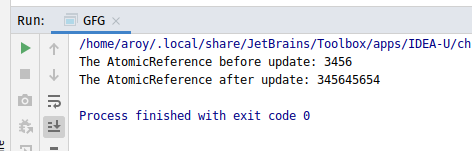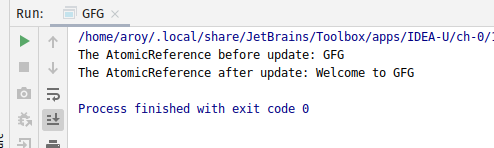# AtomicReference accumulateAndGet() method in Java with Examples

• Last Updated : 03 Jan, 2020

The accumulateAndGet() method of a AtomicReference class is used to atomically updates the current value of AtomicReference with the results of applying the given accumulatorFunction to the current and given values and returns the updated value. The accumulatorFunction should be side-effect-free, since it may be re-applied when attempted updates fail due to contention among threads.

Syntax:

```public final E accumulateAndGet(E x,
BinaryOperator<E> accumulatorFunction)

```

Parameters: This method accepts:

• x which is the updated value and
• accumulatorFunction which is a side-effect-free function of two arguments.

Return value: This method returns the updated value.

Below programs illustrate the accumulateAndGet() method:
Program 1:

 `// Java program to demonstrate``// AtomicReference.accumulateAndGet() method`` ` `import` `java.util.concurrent.atomic.AtomicReference;``import` `java.util.function.BinaryOperator;`` ` `public` `class` `GFG {``    ``public` `static` `void` `main(String args[])``    ``{`` ` `        ``// AtomicReference with value``        ``AtomicReference ref``            ``= ``new` `AtomicReference<>(``3456``);`` ` `        ``// Print AtomicReference``        ``System.out.println(``            ``"The AtomicReference before update: "``            ``+ ref);`` ` `        ``// Value to apply accumulateAndGet``        ``int` `x = ``45654``;`` ` `        ``// Declaring the accumulatorFunction``        ``// applying function to add value as string``        ``BinaryOperator add``            ``= (u, v) -> u.toString() + v.toString();`` ` `        ``// apply accumulateAndGet()``        ``ref.accumulateAndGet(x, add);`` ` `        ``// print AtomicReference``        ``System.out.println(``            ``"The AtomicReference after update: "``            ``+ ref);``    ``}``}`

Output:Program 2:

 `// Java program to demonstrate``// AtomicReference.accumulateAndGet() method`` ` `import` `java.util.concurrent.atomic.AtomicReference;``import` `java.util.function.BinaryOperator;`` ` `public` `class` `GFG {``    ``public` `static` `void` `main(String args[])``    ``{``        ``// AtomicReference with value``        ``AtomicReference ref``            ``= ``new` `AtomicReference(``"GFG "``);`` ` `        ``// Print AtomicReference``        ``System.out.println(``"The AtomicReference before update: "``                           ``+ ref);`` ` `        ``// Value to apply accumulateAndGet``        ``String x = ``"Welcome"``;`` ` `        ``// Declaring the accumulatorFunction``        ``// applying function to add value as string``        ``BinaryOperator add``            ``= (u, v) -> v + ``" to "` `+ u;`` ` `        ``// apply accumulateAndGet()``        ``ref.accumulateAndGet(x, add);`` ` `        ``// print AtomicReference``        ``System.out.println(``            ``"The AtomicReference after update: "``            ``+ ref);``    ``}``}`

Output:My Personal Notes arrow_drop_up